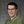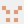0%
0%

# A new derivation of the Gompertz/logistic function

Providing a possible biological substantiation of the Gompertz and logistic function when used in relation to tumor growth

Similar projects worth following
2.2k views
The Gompertz and logistic function in oncology is a popular method for modelling the empirical growth curves of avascular and vascular tumors in the early stage. However, these phenomenological models are purely descriptive and biological vindication is missing. The purpose of this article is to provide possible biological substantiation of the Gompertz and logistic function when used in relation to tumor growth.

In order to answer this question, we will first assume a spherical tumor. Furthermore, the exchange of substances must take place over its surface as for cells. If V is the sphere volume, A is the sphere surface and r is the sphere radius,

applies, which can be demonstrated easily:

A well-known tumor model is the Mendelsohn model  with the tumor volume V(t) and the proportionality constant a:

The Mendelsohn model describes unlimited growth. However, a tumor in the early stage has a sigmoid growth curve, meaning that we cannot follow this model further, but must make a modification by approximating the A/V-Ratio. We will use the following series representation (without proof)

with the domain

for this. By the ratio test, the series (3) converges.

If we stop developing the series at n = 1, we obtain

We define

K will always be designated as capacity below. Therefore, our new model is

This is the Gompertz equation after the growth model of the same name.

A general differential equation can be obtained from (3):

For i = 0 we obtain the exponential growth law, for i = 1 the Gompertz growth law and for i = ∞ the Mendelsohn model. More sigmoid functions can be generated if i is odd.

A second series representation of x^(-k) (without proof) is given by

Let the same domain as for the series representation (3) apply, then the series (7) converges by the ratio test when |1 - x| < 1.

We therefore obtain a further general differential equation:

The result for i = 1 is the Bernoulli differential equation of the logistic growth model. We define

which immediately results in the following:

For i = 0 we obtain again the exponential growth law and for i = ∞ the Mendelsohn model. Also here more sigmoid functions can be generated if we choose i accordingly.

A third series representation of x^(-k) (again without proof) should also be mentioned for reasons of completeness:

again with the same domain as series representation (3) and (7). By the ratio test, the series (10) converges also.

In the same way, we obtain the general differential equation for this:

For i = 0 we obtain linear growth law, for i = ∞ we of course obtain the Mendelsohn model and for i = 1

with the solution

whereby C represents the integration constant and Ei the integral exponential function.

## References

### From the Mendelsohn model to the Gompertz and logistic growth law.pdf

Extended English version of my work in LaTeX

Adobe Portable Document Format - 266.06 kB - 10/01/2016 at 14:11

Extended German version of my work in LaTeX

Adobe Portable Document Format - 146.16 kB - 08/31/2016 at 16:28

• ### Discussion

M. Bindhammer08/21/2016 at 18:56 0 comments

The derived models suggest that the tumor could follow the Mendelsohn model as long as this strategy is optimal. I still have no clue, how long this strategy is optimal. But for sure the tumor has a kind of survival strategy. First the tumor just uptake nutrients by its surface. It is still not detectable by medical exams. Later the tumor changes its strategy, building own blood vessels. This might have two reasons. Reason 1 is that the tumor can't uptake enough nutrients by its surface anymore. Reason 2 is that the tumor, which getting now detectable, has to suspect that it get detected and killed. Therefore the tumor spread (metastases) to maximize the chance that its species survive. Tumor growth could be seen as an evolutionary process, thus extremely optimized and difficult to handle.

Hence:

• Develop a method to detect the tumor as early as possible
• As the tumor is still in the diffusion limited state, do not use ordinary chemotherapeutic agents injected into your bloodstream. Better develop a chemotherapeutic agent which works only locally, similar like radiation therapy or local anesthetic.

More about that in the next log(s).

Share

## Discussions

David H Haffner Sr wrote 07/22/2016 at 16:42 point

This is very facinating work here!

Are you sure? yes | no

M. Bindhammer wrote 07/22/2016 at 19:03 point

Thank you, Sir!

Are you sure? yes | no

M. Bindhammer wrote 06/18/2016 at 17:25 point

Thanks, Rodrigo. Appreciate it!

Are you sure? yes | no

Rodrigo Loza wrote 06/18/2016 at 02:17 point

Very nice model

Are you sure? yes | no

## Similar Projects

Project Owner Contributor

### Flex Test Alignment JigsPatrick

Project Owner Contributor

### QuadRotor testBed for localization and mappingint-smart

# Does this project spark your interest?

Become a member to follow this project and never miss any updates